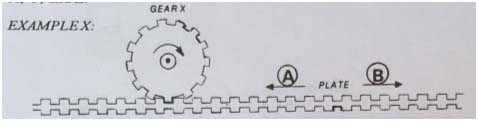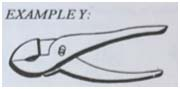Select Page

## MA Test

(Instructions)

In this test, you will answer questions about mechanical facts and principles, by clicking one of the five choices given. Sometimes there will be a picture with the question; sometimes not. Some examples follow. Click the correct answers in the boxes for Examples X, Y, Z.If gear X is locked in its place, but can turn, and is turning in the direction of the arrow, which way is the Plate moving? a. A b. B c. First A, then B d. first B, then A e. the Plate is not movingWhich is this tool? a. wrench b. pipe wrench c. scissors d. pliers e. clamp
 EXAMPLE Z: Any material that electricity will pass though is called a(n) a. Insulator b. magnet c. transformer d. filament e. conductor

The correct answer for EXAMPLE X is a., since the plate would have to move in direction A. For Example Y, the answer is d. pliers, and for EXAMPLE Z, the answer is e. conductor. Therefore, you should have clicked in a. box for EXAMPLE X, the d box for EXAMPLE Y and e. box for EXAMPLE Z.

Work as quickly as you can. You will have 9 minutes for all the questions on this test. If you are not sure of the right answer for a question, click the choice that is your best guess.

Click the button below to proceed